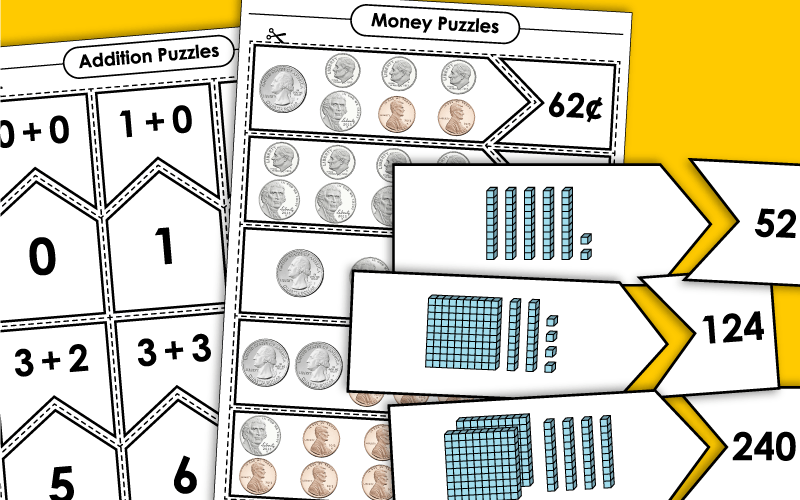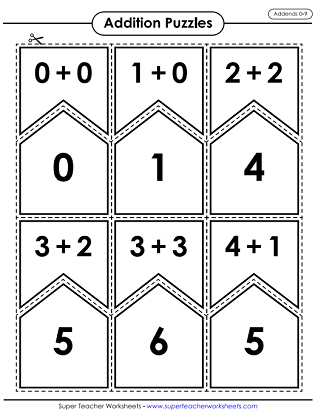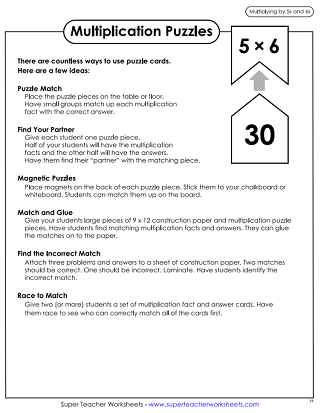# Puzzle Match Math Games

There are many ways to use these printable puzzle pieces in your classroom. Cut apart the puzzle pieces and students can match math facts with the answers. We have puzzles for addition, subtraction, multiplication, division, time, money and more! Use them in math learning centers or small group activities.Students can practice their basic addition facts with this printable puzzle game.
Print and cut these puzzle pieces to practice counting coins. Match each coin set with the correct money amount.
Practice counting coins with this printable puzzle. Match money amounts with coin sets.
Use these printable puzzle pieces to practice basic division facts. Divisors up to 9, and dividends up to 81.
Print and cut out these puzzle pieces so students can practice their basic multiplication facts.
Students can practice their basic multiplication facts (factors 0-12) using this printable activity.
Print and cut these puzzle pieces so students can practice multiplying by 0s, 1s and 2s.
Practice multiplying by 3s and 4s with this fun printable puzzle activity.
Brush up on your multiplication skills using this printable resource. Problems include multiplying by 5s and 6s.
Students can use this printable puzzle resource to practice multiplying by 7s and 8s.
Review your multiplication skills with this printable activity that focuses on multiplying by 9s and 10s.
Practice multiplying by 11s and 12s with this fun activity. Print and cut the puzzle pieces.
Each number is represented with base ten blocks. Find the correct number matches to practice tens and ones place value.
Match each number with the base ten blocks that represent it. Numbers go up to the hundreds place.
This printable puzzle has pieces with numbers up to the thousands place. Match them with their correct base ten models to complete the game.
Print these puzzle pieces to match each number with its expanded form.
Use this printable puzzle to brush up on your place value. Match each number with it's expanded form up to the hundred thousands place.
These printable puzzle pieces have numbers up to the millions place. Match each number with the correct expanded form.
Print out this puzzle to practice basic subtraction. Minuends and subtrahends up to 9.
Print out these puzzle pieces to practice basic subtraction.

## English -Language Arts Puzzle Match

Use this printable puzzle to match common contractions with the words they represent.

## Social Studies Puzzle Match

There are countless ways to use this printable puzzle. Match each Canadian province/territory with its capital.
Print this puzzle to match each state with its capital.
Scoot Classroom Game

Scoot is another printable math card game you can play with your whole class.

More Math Worksheets

We have thousands of math worksheets and games to use in your classroom!

## Worksheet ImagesMy Account
Site Information